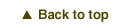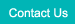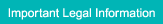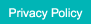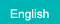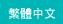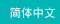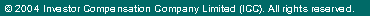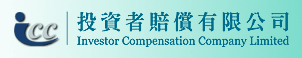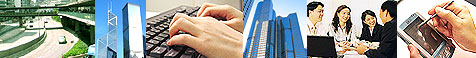Calculation of the Compensation AmountMr. Wong trades Hang Seng Index ("HSI") futures contracts: Mr. Wong bought 8 HSI futures contracts when HSI was 27,000 and the margin required is HK\$800,000 (assume HK\$100,000 per HSI futures contracts) Assume ABC Brokerage Firm defaults on 2/1/202X, then the closing HSI on the default date is used to calculate the compensation amount: HSI closes at 26,700 on 2/1/202X Each contract losses HK\$15,000 (HK\$50 for each index point) and total loss is HK\$120,000 for 8 contracts The balance of the futures contract margin is HK\$680,000 as at the default date.

Mr. Wong should receive a compensation amount of HK\$500,000.

A claimant would receive a maximum compensation amount of HK\$500,000.